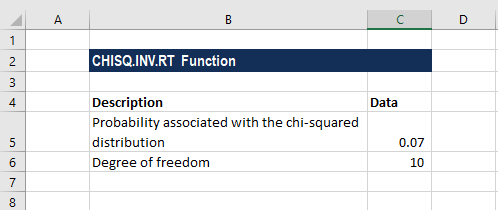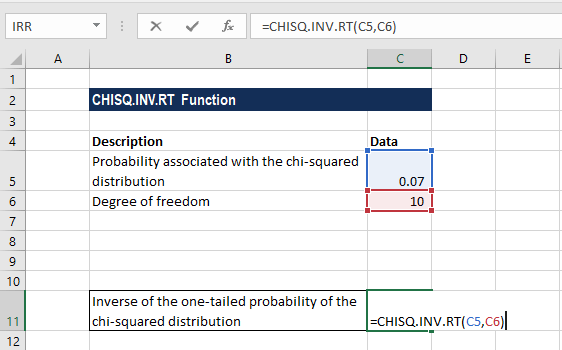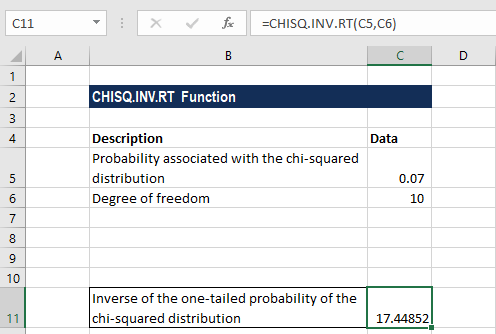# CHISQ.INV.RT Function

Calculates the inverse of the right-tailed probability of a chi-square distribution

## What is the CHISQ.INV.RT Function?

The CHISQ.INV.RT Function is categorized under Excel Statistical functions. It will calculate the inverse of the right-tailed probability of a chi-square distribution. We use this function to compare observed and expected values.

In financial analysis, the CHISQ.INV.RT function can be used to find out and compare observed and expected values. For example, a genetic experiment might hypothesize that the next generation of plants bearing fruits will exhibit a certain set of traits. By comparing the observed results with the expected ones, we can decide whether our hypothesis is valid or not.

### Formula

=CHISQ.INV.RT(probabilty,deg_freedom)

The CHISQ.INV.RT function uses the following arguments:

1. Probability (required argument) – This is the probability of the right-tailed chi-square distribution, for which we want to find the value of x.
2. Deg_freedom (required argument) – This is the number of degree of freedoms. It must be a positive integer.

So, for a supplied probability, the CHISQ.INV.RT function finds the value of x such that CHISQ.DIST.RT(x,degrees_freedom) = probability. Hence, the precision of CHISQ.INV.RT depends on the precision of CHISQ.DIST.RT.

### How to use the CHISQ.INV.RT Function in Excel?

To understand the uses of the CHISQ.INV.RT function, let us consider an example:

#### Example

Suppose we are given the following data with us:The formula for calculating the one-tailed probability of the chi-squared distribution is:We get the result below:### A few notes about the CHISQ.INV.RT Function

1. If the deg_freedom argument is not an integer, it is truncated by MS Excel.
2. The CHISQ.INV.RT function belongs to the CHISQ family of functions. The function was introduced in Excel 2010 and hence is unavailable in earlier versions. It is an updated version of the CHIINV function.
3. #VALUE! error – Occurs when any of the arguments provided is non-numeric. Remember, the function will accept only numeric data sets for both arguments and return numeric data.
4. #N/A error – CHISQ.INV.RT uses an iterative search technique. If the search is not converged after 64 iterations, the function will return this error.
5. #NUM! Error – Occurs when:
1. The given value of probability is less than or equal to 0 or is greater than 1.
2. The deg_freedom argument is less than 1.

CFI is the official provider of the Financial Modeling Analyst Certification, designed to transform anyone into a world-class financial analyst.

Thanks for reading this guide to important Excel functions! By taking the time to learn and master these functions, you’ll significantly speed up your financial analysis. To learn more, check out these additional CFI resources:

• Excel Functions for Finance